# How to Calculate and Solve for the Length and Perimeter of a Rhombus | Nickzom Calculator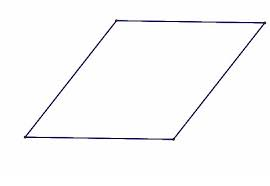The image above is a rhombus.

To compute the area of a rhombus, one essential parameter is needed and this parameter is length of side (a).

The formula for calculating the perimeter of a rhombus:

P = 4a

Where;
P = Perimeter of the rhombus
a = length of side

Let’s solve an example;
Find the perimeter of a rhombus when the length of side is 30 cm.

This implies that;
a = Length of side = 30 cm

P = 4a
P = 4 x 30
P = 120

Therefore, the perimeter of the rhombus is 120 cm.

Calculating the Length of Side using the Perimeter of the Rhombus.

a = P / 4

Where;
a = Length of Side
P = Perimeter of the rhombus

Let’s solve an example;
Given that the perimeter of the rhombus is 180 cm. Find the length of side?

a = P / 4

This implies that;
P = perimeter of the rhombus = 180 cm

a = P / 4
a = 180 / 4
a = 45

Therefore, the length of side is 45 cm.

Nickzom Calculator – The Calculator Encyclopedia is capable of calculating the perimeter of a rhombus.

To get the answer and workings of the perimeter of a rhombus using the Nickzom Calculator – The Calculator Encyclopedia. First, you need to obtain the app.

You can get this app via any of these means:

To get access to the professional version via web, you need to register and subscribe for NGN 1,500 per annum to have utter access to all functionalities.
You can also try the demo version via https://www.nickzom.org/calculator

Once, you have obtained the calculator encyclopedia app, proceed to the Calculator Map, then click on Mensuration under the Mathematics section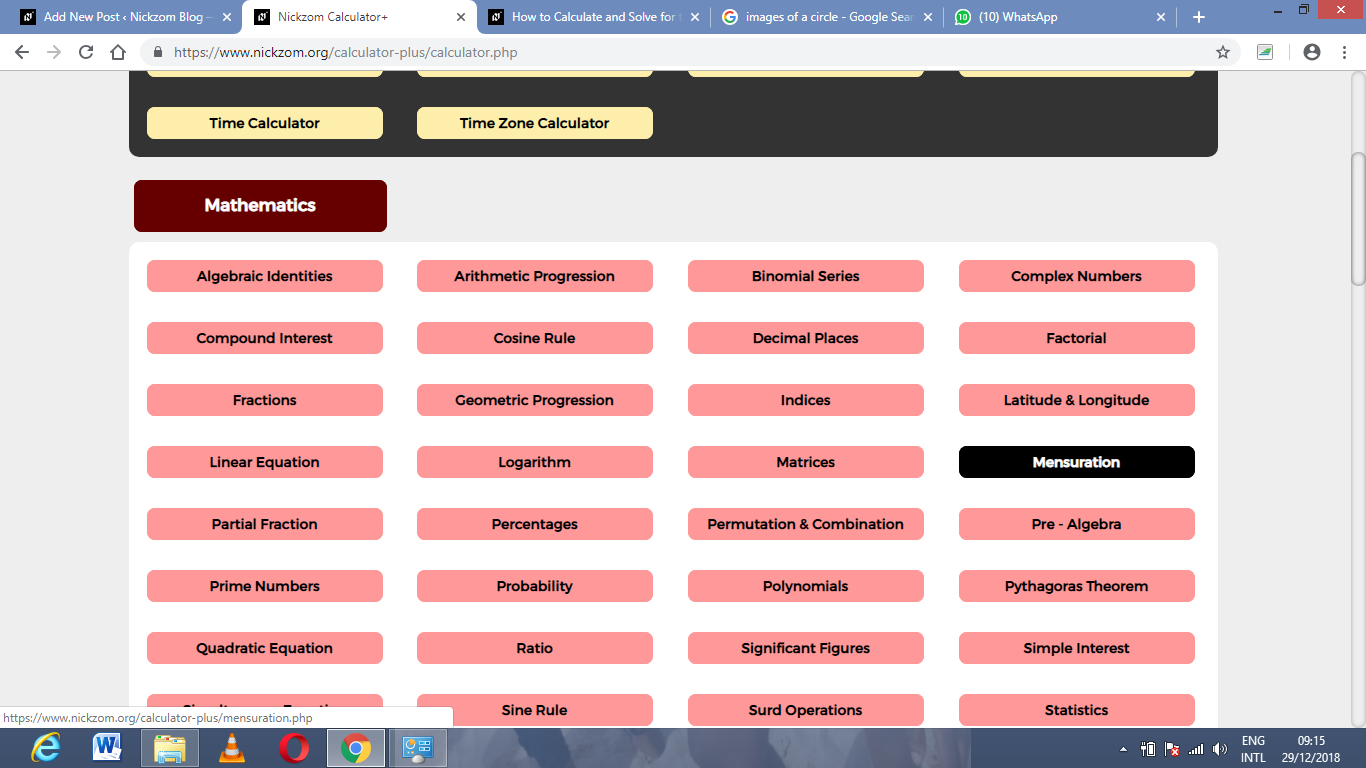Now, click on Perimeter of a Rhombus under MensurationThe screenshot below displays the page or activity to enter your value, to get the answer for the perimeter of a rhombus according to the respective parameter which is the length of side (a).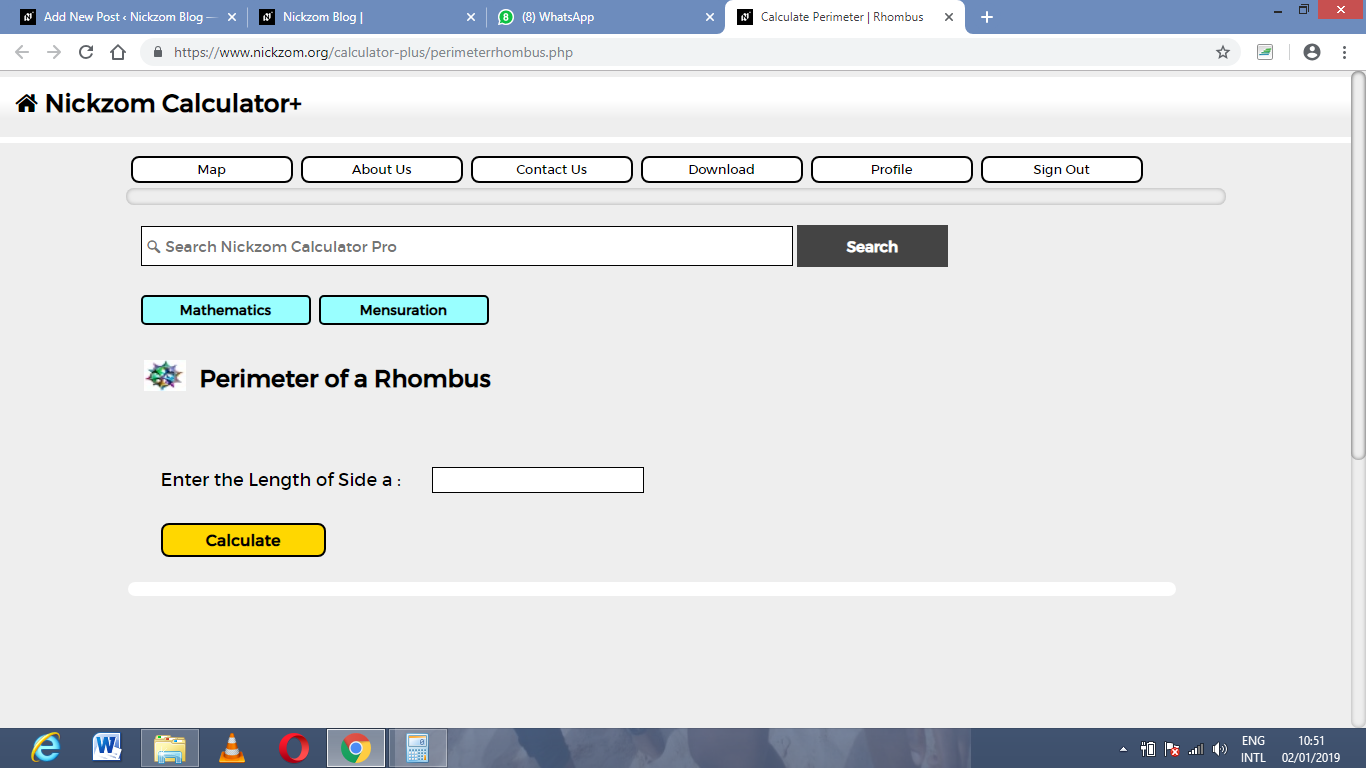Now, enter the values appropriately and accordingly for the parameter as required by the example above where the length of side (a) is 19 cm.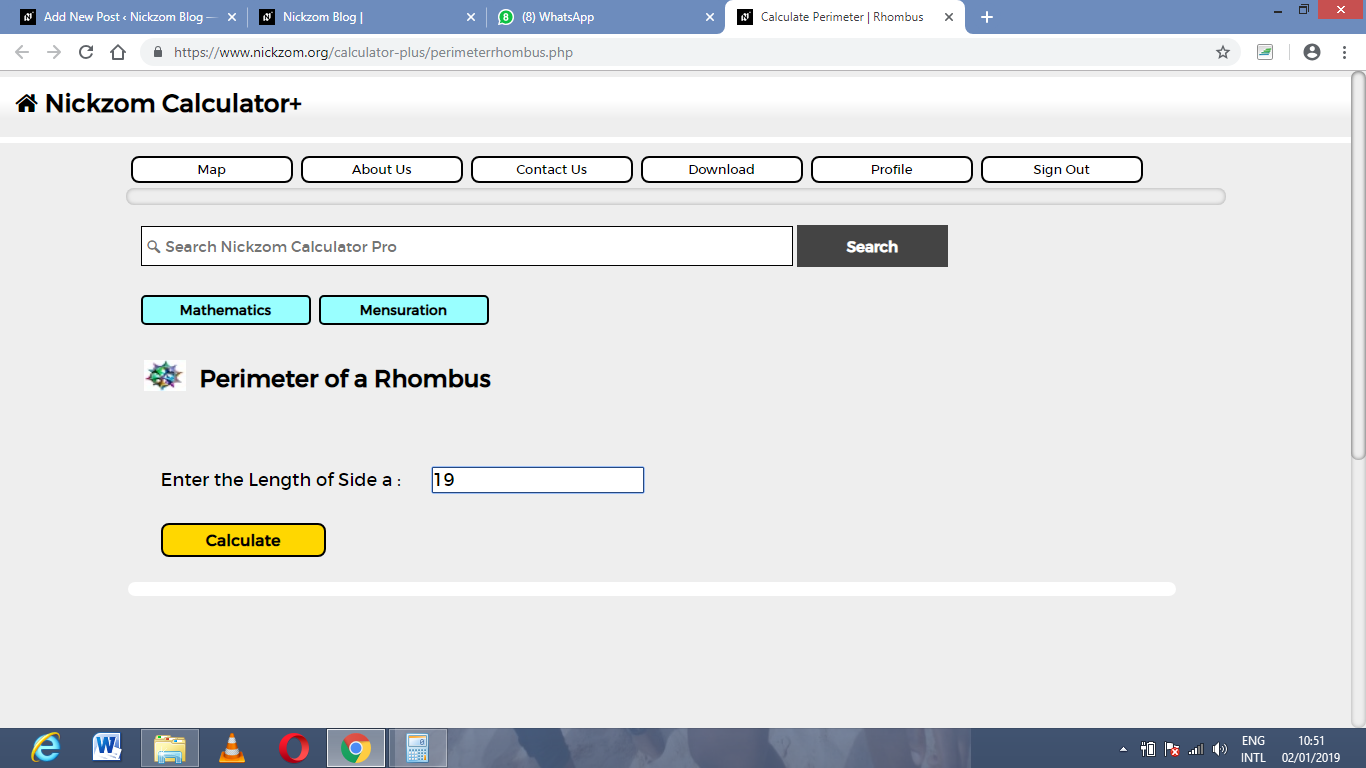Finally, Click on Calculate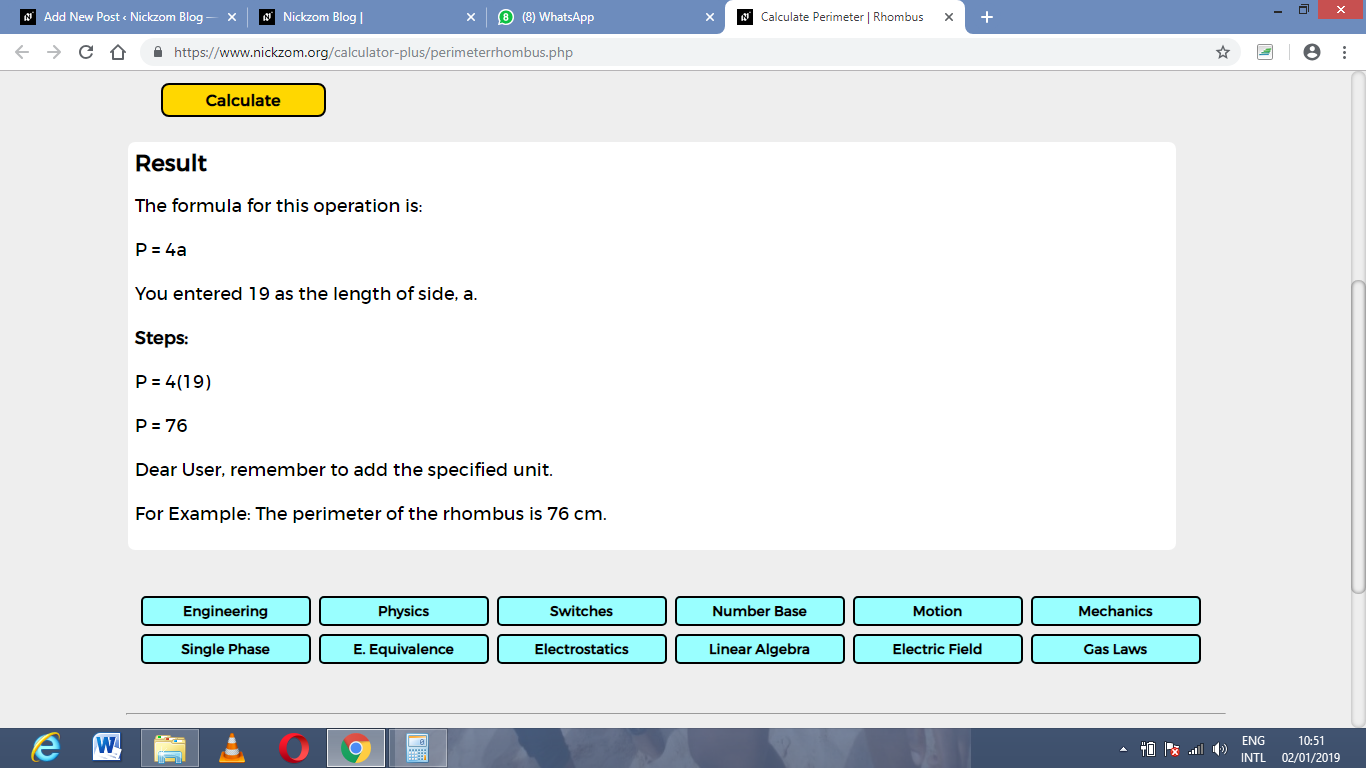As you can see from the screenshot above, Nickzom Calculator – The Calculator Encyclopedia solves for the perimeter of a rhombus and presents the formula, workings and steps too.